# Saxon Math Intermediate SeriesSaxon Math already has a series of books for kindergarten through third grade, and another series that begins with Math 5/4 for fourth grade and continues up through Calculus. So why this intermediate series for grades three, four, and five? For third grade, at least part of the answer lies in the differences between the Saxon Math program for K-3 and the rest of the Saxon books from Math 5/4 up through Calculus. The K-3 program is completely different from the other Saxon books. They were written by a different author, incorporate manipulatives, and have a very teacher-intensive form of lesson presentation.

Math 5/4 and all the texts beyond were written by John Saxon, Stephen Hake, or both of them together. Saxon Math Intermediate 3, 4, and 5 were written by Stephen Hake and, consequently, are more similar to the upper-level books than to the K-3 series. Those just starting into Saxon Math at third-grade level should probably go with Math Intermediate 3 since it ensures a more familiar transition into either Math Intermediate 4 or Math 5/4. Those who have been using the Saxon K-3 series up through second grade might go either way, perhaps deciding based upon a preference for manipulatives used in the K-3 courses or a preference for the less teacher-intensive Intermediate 3 course. For older students, you need to choose between Math Intermediate 5 and Math 6/5.

Most of the content remains very similar in scope and sequence to the rest of the Saxon math program, retaining Saxon’s incremental, spiral approach of introducing a concept or skill, then expanding upon it in one or more future lessons.  Those future lessons are usually spaced out, showing up weeks later rather than being taught consecutively. Practice problems review concepts, and they also present those concepts in different fashions (e.g., traditional mathematical expressions and word problems) rather than presenting arrays of similar problems. For some students, this strengthens their thinking ability and keeps things interesting while for others it’s just confusing.

The Saxon Math Intermediate courses differ some from the Math 54 and up courses in layout, and they require a little more teacher involvement. Parents might need to work with students through the "Power Up" activities described below and the presentation of new concepts.

There are four components to the homeschool kit for each level: the student text, a solutions manual, a Power Up Workbook, and a Homeschool Testing Book.

Each lesson begins with “Power Up” activities. These are a bit more involved than the “Warm Up” activities that begin each lesson in Math 5/4 and Math 6/5. Power Up activities include four categories of problems each time: math fact review, count aloud or jump start, mental math, and problem solving. (Math Intermediate 3 labels the second category “jump start” while Intermediate 4 and 5 label it "count aloud.") This second category might include oral counting by increments, drawing hands on a clock, thermometer reading, writing a fact family for three numbers, etc. The count aloud category is dropped from some of the Power Up sections in Math Intermediate 4 and 5 since by fourth and fifth grades students should be able to skip count fairly proficiently and will need only occasional review. The separate Power Up Workbook is used for the written activities for the Power Up section with one page per lesson. The top half of each page is for math fact review problems. Then sections for each of the other three types of activities might each contain some content (e.g., clock face in jump start) or simply provide blank space for students to write their responses. Some students will need assistance in completing these activities, especially at the beginning of the school year.

After the Power Up activities, a new concept is introduced along with sample problems that are completely worked out. The series includes some work on conceptual understanding as new skills and concepts are taught. However, concepts are taught with written or pictorial explanations rather than manipulatives (aside from fraction manipulatives used a few times in fourth grade), and conceptual development is not as strong as in Singapore Math or Math-U-See. After the new concept is presented, some lesson practice problems provide students with practice only on the new concept. “Written Practice” problems follow with 15 to 20 problems per lesson for third grade that review previously taught concepts. The number of Written Practice problems increases to 30 per lesson in fourth and fifth grades. The Written Practice problems are wide-ranging in approach, really challenging students’ thinking skills as is done in Singapore Math. Occasionally, students encounter an open-ended question that asks for both an answer and an explanation. Some lessons add an extra problem for “Early Finishers.” These are usually word problems that make real-world connections that are either more time-consuming or more challenging than the other lesson problems. These aren’t the only real-world word problems, since lessons generally have quite a few of them.

Students will need to work in a notebook or on other paper to complete lesson practice and Written Practice problems. They will not be writing in the hardcover textbook.

After every ten lessons there is an Investigation lesson. Each of these will likely take an entire class period. For example, one Investigation in Math Intermediate 3 teaches about bar graphs then directs students to collect survey data by asking questions then create their own bar graph. Investigations will require parental/teacher oversight or direction.

Instead of a teacher manual, the Solutions Manual for each course has answers and complete solutions (when applicable) for all textbook problems. There is no need for a teacher’s manual in addition to this since the text is self-explanatory. There is no answer key for the Power Up Workbook, although parents/teachers should be able to check the answers fairly easily without a key. (It appears that there is a CD with a Power Up answer key available in the school edition for each course but which has been omitted from the homeschool kits.)

The Homeschool Testing Book for each course has tests that follow every fifth lesson beginning after the tenth lesson, plus answer keys for all tests. Since tests are cumulative, the Test Analysis Form in this book is useful for identifying the lessons where tested concepts were originally taught in case a student needs to review that lesson.

The Math Intermediate series is totally in alignment with the Common Core Standards. On the publishers website they identify which lessons meet each of the standards for each grade level. Math Intermediate 4 and Math Intermediate 5 are very similar to Math 5/4 and Math 6/5 respectively in their content with some identical material.

However, there are some additional or expanded topics in the Math Intermediate texts. For example, graphing points on a coordinate plane is introduced in an Investigation in Math Intermediate 4 but is not taught at all in Math 5/4. Reciprocal fractions are taught in one lesson in Math 6/5 while they show up in four lessons in Math Intermediate 5. Roman numerals were also added to Math Intermediate 5 in an appendix.

Note that all three Math Intermediate texts refer occasionally to Lesson Activity worksheets that are not found in the homeschool package. These are not essential.

Math Intermediate 3 covers addition and subtraction review, multiplication through one-digit multipliers times three-digit multiplicands, division through one-digit divisors into two-digit dividends, measurement, rounding, estimation, number concepts, geometric shapes, area, perimeter, time, money, calendar reading, graphing, and probability.

Math Intermediate 4 reviews addition, subtraction, and number concepts. Then it continues through multiplication and division of whole numbers. It also teaches fractions up through addition and subtraction of fractions and mixed numbers, including those with different denominators. Money is used to introduce decimal numbers. In addition, students study quite a bit of geometry, learn about averages, and work with tables, schedules, and graphs.

Math Intermediate 5 reviews quite a bit of what was taught in Math Intermediate 4, then moves on to multiplication and division of fractions, all four functions with decimal numbers, and an introduction of percents.

Overall, the Math Intermediate series is very easy to use and allows students to do much of their work independently, so it should work well in many homeschooling situations. However, students who need to stay focused on one concept at a time might not do as well with this incremental approach and the mixed practice problems.

### Pricing Information

When comparison prices appear, please keep in mind that they are subject to change. Click on links where available to verify price accuracy.

#### Saxon Math Intermediate 3: Written Practice Workbook 1st Edition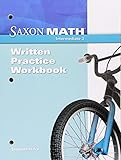#### Saxon Math Power-Up Workbook: Intermediate 3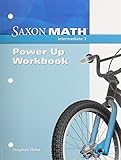#### Saxon Math Intermediate 3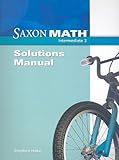#### Saxon Math Intermediate 3 Homeschool Testing Book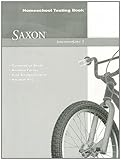#### Saxon Math Intermediate 3: Complete Kit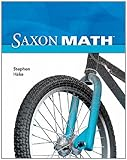#### Saxon Homeschool Intermediate 4: Homeschool Package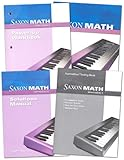#### Saxon Math Intermediate 5 Comp Homeschool Kit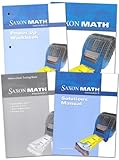Get a FREE subscription to Cathy's E-Newsletter

### My Lists

Save reviews to lists to guide curriculum choices. Register or log in to start your first list.

### Instant Key

• Need For Parent or Teacher Instruction: low to moderate
• Learning Environment: group or one-on-one
• Educational Methods: spiral and incremental, lots of variety, interactive, critical thinking